# CTC理论和实战

Posted by lili on

## CTC简介

• X和Y都是变长的
• X和Y的长度比也是变化的(X和Y的长度不存在简单的比例对应关系)
• 训练数据中没有X和Y的对齐

CTC可以解决这些问题，给定一个输入X，CTC可以对所有可能的Y计算$P(Y \vert X)$。有了这个概率，我们就可以推断最可能的输出或者计算某个Y的概率。在训练的时候，我们需要计算损失函数，并且通过梯度下降调整参数使得损失在训练数据上最小。为了实现训练，我们需要一种高效快速的方法来计算条件概率$P(Y \vert X)$，而且还要求它是可导的，这样我们才能计算梯度。而在预测的时候，给定输入X，我们需要计算最可能的Y：

$Y^*=\underset{Y}{argmax}P(Y|X)$

CTC虽然没有精确的算法来高效的计算最优路径，但是它提供近似的算法使得我们能在合理的时间内找到较优的路径。

## CTC算法详解

### 对齐

CTC算法是不需要对齐输入和输出的，为了计算给定X的条件下输出Y的概率，CTC会枚举所有可能的对齐方式然后把这些概率累积起来。要理解CTC算法，我们首先需要理解对齐。

• 强制要求每个输入都对应实际的输出是不合理的，比如在语音识别中会有静音(silence)，这些输入不对应任何输出
• 没办法输出连续相同的字符，比如假设有一个单词caat，那么上面的对齐只能认为输出是cat。

CTC对齐有如下一些特性。首先它是单调的，如果我们输入往前一步，那么输出可以保持不变，也可以往前一步。比如假设$x_1$是c，与之对于的y是c，我们往前走一步，有可能$x_2$还是c，那么y的输出是保持不变的。当然也可能$x_2$是a了，那么y的输出也往前走一步变成ca。第二个特点就是输入与输出是多对一的关系，输出的c可以对于$x_1,x_2$两个输入，但一个输入$x_1$只能对应一个输出。这个特性可以推出如下结论：输入序列的长度一定是大于等于输出长度的。

### 损失函数

$\begin{split} P(Y|X)= \underset{A \in \mathcal{A}_{X,Y}}{\sum} \prod_{t=1}^{T}p_t(a_t|X) \\ \end{split}$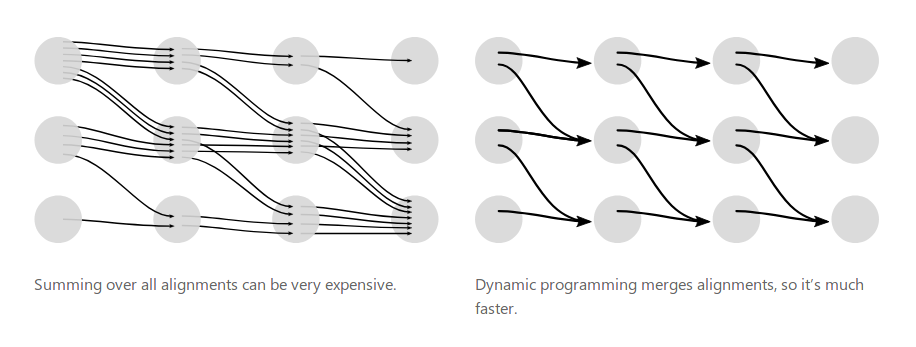图：CTC的穷举和动态规划算法

$\alpha_{s,t}=(\alpha_{s-1,t-1} + \alpha_{s, t-1}) \cdot p_t(z_s|X)$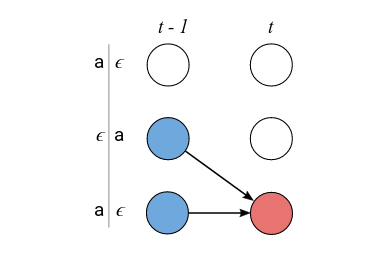图：不能跳过$z_{s-1}$的情况

$\alpha_{s,t}=(\alpha_{s-2,t-1} + \alpha_{s-1,t-1} + \alpha_{s, t-1}) \cdot p_t(z_s|X)$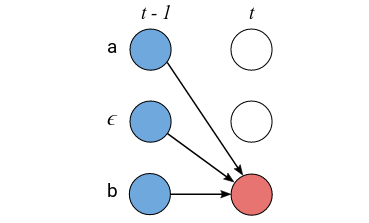图：能跳过$z_{s-1}$的情况

$L=\underset{(X,Y) \in \mathcal{D}}{\sum}-log P(Y|X)$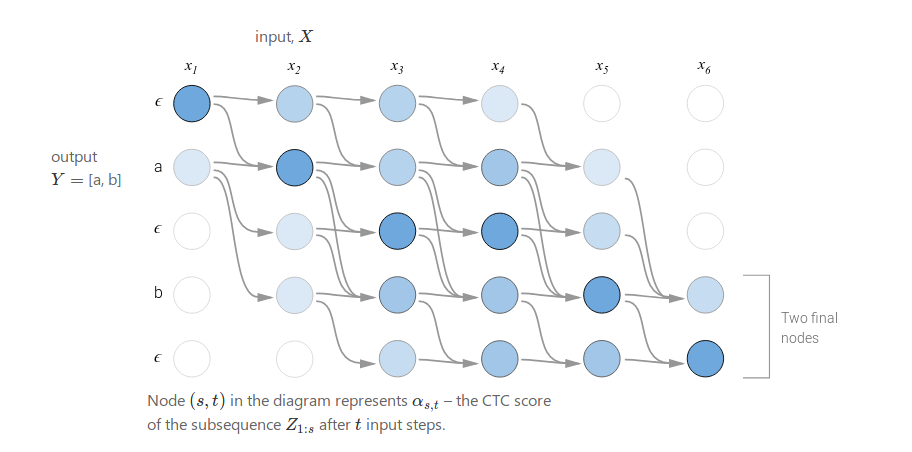图：动态规划计算$\alpha$的过程

### 预测

$Y^*=\underset{Y}{argmax}P(Y|X)$

$A^*=\underset{A}{argmax}\prod_{t=1}^{T}p_t(a_t|X)$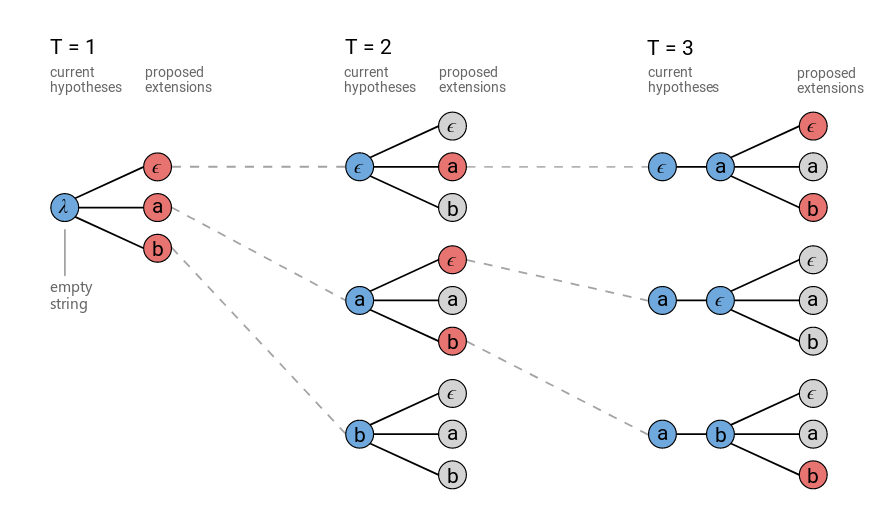图：普通的Beam Search算法，字母表为${a,b,\epsilon}$，beam大小是3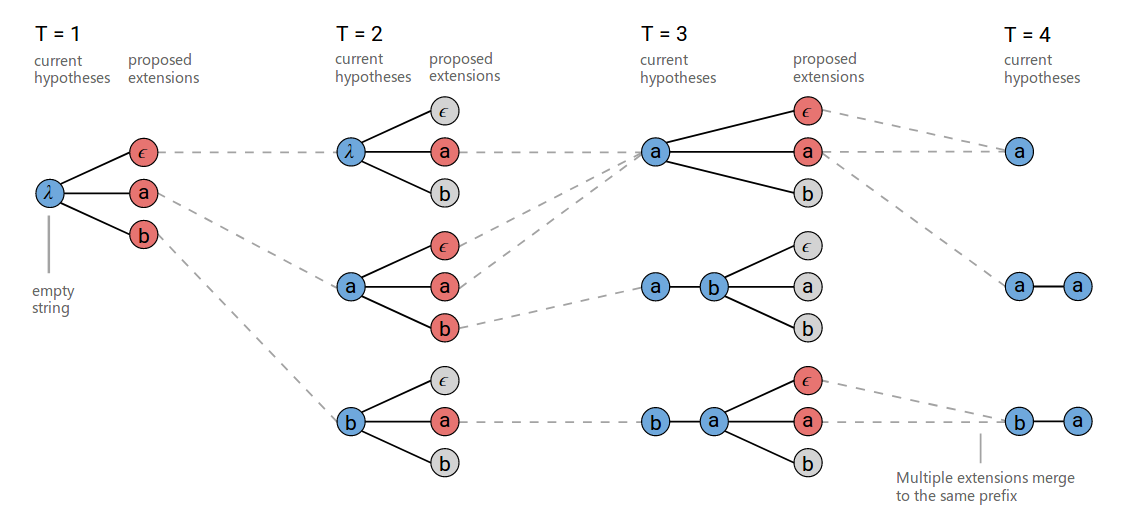图：改进的Beam Search算法，字母表为${a,b,\epsilon}$，beam大小是3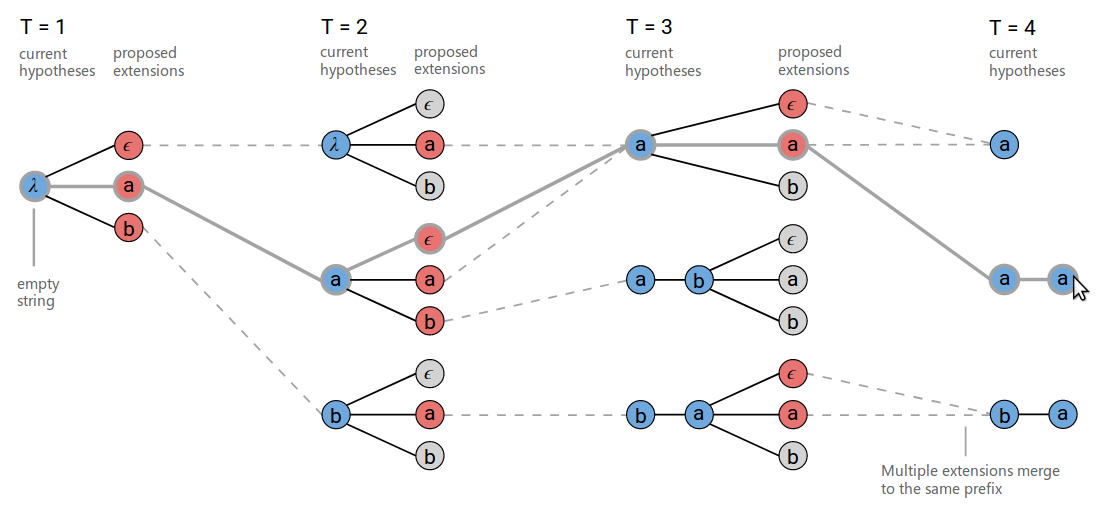图：注意鼠标选中的aa的粗线条路径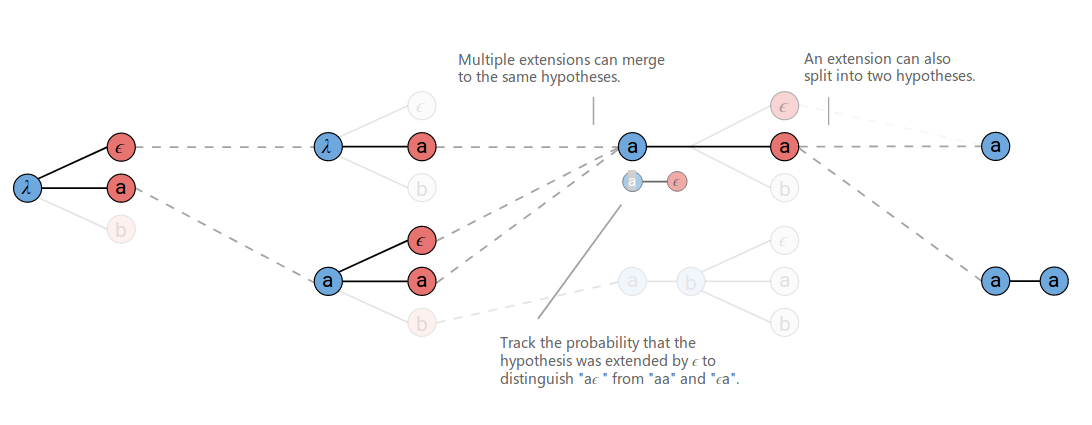图：在合并的时候记录是否有空结尾的路径以及它们的概率和

$Y^*=\underset{Y}{argmax}p(Y|X) \cdot p(Y)^\alpha \cdot L(Y)^\beta$

$P(Y)$就是语言模型，而$L(Y)$是一个语言模型长度的奖励，如果$L(Y)$是基于词的语言模型，那么$L(Y)$就是词的个数，如果$L(Y)$是基于字符(character)的语言模型，那么$L(Y)$就是字符的个数。因为越长的句子概率越小，如果不加这个奖励的话，语言模型总是会倾向于选择短的句子。超参数$\alpha$和$\beta$通常通过交叉验证来选择。

## CTC算法的特性

### 条件独立

CTC经常被诟病的一个特点就是它的条件独立性。这个模型假设给定X的时候不同时刻的$y_t$是独立的，这个假设对于很多序列标注问题来说是不合理的。假设一个语音是要说”三个A“，它有两种说法：”三个A(triple A)”;”AAA”。如果第一个输出是A，那么第二个输出A的概率应该变大；类似的如果第一个输出是t（英文的triple的t)，那么输出triple A的概率更大，如下图所示。但是CTC无法建模这种关系。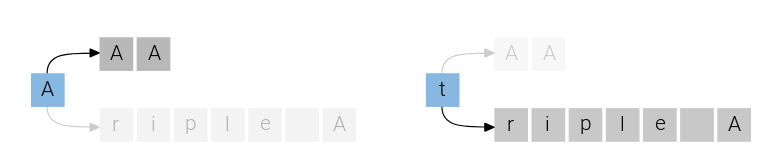图：triple A和AAA

## 对齐

CTC算法不需要训练数据对齐，它会把所有相同输出的对齐合并。虽然CTC要求输入X和输出Y严格对齐，但是具体怎么对齐它并没有在模型层面加任何限制，是把概率比较均匀的分配给所有可能的路径还是把概率集中的分配给某些路径，这是不能确定的。

CTC要求对齐的方式是单调的，这对于语音识别是合适的假设，但是对于其它的任务，比如机器翻译，这种对齐是不合适的。因为一个不同语言的语序是不同的，比如英语a friend of mine和我的朋友，在英语里，friend在mine之前，但是在汉语里”我的”在”朋友”之前。

CTC的另外一个要求就是输入和输出是多对一的，有的任务可以要求严格的一对一关系，比如词性标注，那CTC也是不合适的。另外它也无法表示输入与输出的多对一的关系。比如在英语中，th是一个音素，一个输入可能要对于th这两个输出，CTC也是无法表示这种关系的。

## 使用CTC进行变长验证码识别

### 安装WarpCTC

#### 得到tensorflow源代码

git clone https://github.com/tensorflow/tensorflow.git
git checkout r1.6.0


#### 设置环境变量TENSORFLOW_SRC_PATH

#export TENSORFLOW_SRC_PATH=/path/to/tensorflow


#### 修改配置

lili@lili-Precision-7720:~/codes/warp-ctc$git diff CMakeLists.txt diff --git a/CMakeLists.txt b/CMakeLists.txt index cdb4b3e..ec20845 100644 --- a/CMakeLists.txt +++ b/CMakeLists.txt @@ -6,6 +6,7 @@ ENDIF() project(ctc_release) +set(CMAKE_CXX_FLAGS "${CMAKE_CXX_FLAGS} -D_GLIBCXX_USE_CXX11_ABI=0 -L /home/lili/py3-env/lib/python3.5/site-packages/tensorflow -ltensorflow_framework")



lili@lili-Precision-7720:~/codes/warp-ctc\$ git diff tensorflow_binding/setup.py

...

--- a/tensorflow_binding/setup.py
+++ b/tensorflow_binding/setup.py
@@ -52,11 +52,13 @@ root_path = os.path.realpath(os.path.dirname(__file__))

tf_include = tf.sysconfig.get_include()
tf_src_dir = os.environ["TENSORFLOW_SRC_PATH"]
-tf_includes = [tf_include, tf_src_dir]
+tf_includes = [tf_include, tf_src_dir, ("/home/lili/py3-env/lib/python3.5/site-packages/tensorflow/include/external/nsync/public")]
...
extra_compile_args = ['-std=c++11', '-fPIC']
+extra_compile_args += [ '-D_GLIBCXX_USE_CXX11_ABI=0']
...

-
+import tensorflow as tf
+TF_LIB=tf.sysconfig.get_lib()
+print(TF_LIB)
ext = setuptools.Extension('warpctc_tensorflow.kernels',
sources = lib_srcs,
language = 'c++',
include_dirs = include_dirs,
-                           library_dirs = [warp_ctc_path],
+                           library_dirs = [warp_ctc_path, TF_LIB],
runtime_library_dirs = [os.path.realpath(warp_ctc_path)],
-                           libraries = ['warpctc'],
+                           libraries = ['warpctc','tensorflow_framework'],
extra_compile_args = extra_compile_args)



setup.py需要修改3个地方，第一个就是tf_includes里增加nsync_cv.h头文件的路径；第二个就是增加C++选项-D_GLIBCXX_USE_CXX11_ABI=0；第三个就是在setuptools.Extension里增加tensorflow_framework库和它的路径。

#### build

cd warp-ctc
mkdir build; cd build
cmake ..
make


#### 安装

python setup.py install


#### 测试安装是否成功

python
import warpctc_tensorflow


### 运行代码

pip install captcha
git clone https://github.com/fancyerii/lstm_ctc_ocr.git
cd lstm_ctc_ocr
./train.sh


### 代码阅读

#### 数据处理

def generateImg():
if not os.path.exists(cfg.FONT):
print('cannot open the font')
theChars=gen_rand()
return np.array(data),theChars


def groupBatch(imgs,labels):
max_w = -sys.maxsize
time_steps = []
label_len = []
label_vec = []
img_batch = []
nh = cfg.IMG_HEIGHT
for i,img in enumerate(imgs):
if cfg.NCHANNELS==1: h,w = img.shape
else: h,w,_ = img.shape
nw = int(nh/h*w)
max_w = max(max_w,nw)
imgs[i] = cv2.resize(img,(nw,nh))
time_steps.append(nw//cfg.POOL_SCALE+cfg.OFFSET_TIME_STEP) # 这句代码的解释在后面
code = [encode_maps[c] for c in list(labels[i])]
label_vec.extend(code)
label_len.append(len(labels[i]))
max_w = math.ceil(max_w/cfg.POOL_SCALE)*cfg.POOL_SCALE
for img in imgs:
if cfg.NCHANNELS==1: h,w = img.shape
else: h,w,_ = img.shape
img = cv2.copyMakeBorder(img,0,0,0,max_w-w,cv2.BORDER_CONSTANT,value=0).
astype(np.float32)/255.
img = img.swapaxes(0, 1)
img = np.reshape(img,[-1,cfg.NUM_FEATURES])
img_batch.append(img)
return img_batch,label_vec,label_len,time_steps


max_w = math.ceil(max_w/cfg.POOL_SCALE)*cfg.POOL_SCALE


#### network.py

@layer
def conv(self, input, k_h, k_w, c_o, s_h, s_w, name, c_i=None, biased=True,relu=True,

if not c_i: c_i = input.get_shape()[-1]
convolve = lambda i, k: tf.nn.conv2d(i, k, [1, s_h, s_w, 1], padding=padding)
with tf.variable_scope(name) as scope:
init_weights = tf.contrib.layers.xavier_initializer()
init_biases = tf.constant_initializer(0.0)
kernel = self.make_var('weights', [k_h, k_w, c_i, c_o], init_weights, trainable, \
regularizer=self.l2_regularizer(cfg.TRAIN.WEIGHT_DECAY))
if biased:
biases = self.make_var('biases', [c_o], init_biases, trainable)
conv = convolve(input, kernel)
if relu:
return tf.nn.relu(bias)

else:
conv = convolve(input, kernel)
if relu:
return tf.nn.relu(conv)
return conv


def layer(op):
def layer_decorated(self, *args, **kwargs):
# Automatically set a name if not provided.
name = kwargs.setdefault('name', self.get_unique_name(op.__name__))
# Figure out the layer inputs.
if len(self.inputs)==0:
raise RuntimeError('No input variables found for layer %s.'%name)
elif len(self.inputs)==1:
layer_input = self.inputs
else:
layer_input = list(self.inputs)
# Perform the operation and get the output.
layer_output = op(self, layer_input, *args, **kwargs)
self.layers[name] = layer_output
# This output is now the input for the next layer.
self.feed(layer_output)
# Return self for chained calls.
return self
return layer_decorated


#### LSTM_train类

class LSTM_train(Network):
class LSTM_train(Network):
def __init__(self, trainable=True):
self.inputs = []

#N*t_s*features*channels
self.data = tf.placeholder(tf.float32, shape=[None, None, cfg.NUM_FEATURES ], name='data')
self.labels = tf.placeholder(tf.int32,[None],name='labels')
self.time_step_len = tf.placeholder(tf.int32,[None], name='time_step_len')
self.labels_len = tf.placeholder(tf.int32,[None],name='labels_len')

self.keep_prob = tf.placeholder(tf.float32)
self.layers = dict({'data': self.data,'labels':self.labels,
'time_step_len':self.time_step_len,
'labels_len':self.labels_len})
self.trainable = trainable
self.setup()


def setup(self):
(self.feed('data')
.conv_single(3, 3, 64 ,1, 1, name='conv1',c_i=cfg.NCHANNELS)
.max_pool(2, 2, 2, 2, padding='VALID', name='pool1')
.conv_single(3, 3, 128 ,1, 1, name='conv2')
.max_pool(2, 2, 2, 2, padding='VALID', name='pool2')
.conv_single(3, 3, 256 ,1, 1, name='conv3_1')
.conv_single(3, 3, 256 ,1, 1, name='conv3_2')
.max_pool(1, 2, 1, 2, padding='VALID', name='pool2')
.conv_single(3, 3, 512 ,1, 1, name='conv4_1', bn=True)
.conv_single(3, 3, 512 ,1, 1, name='conv4_2', bn=True)
.max_pool(1, 2, 1, 2, padding='VALID', name='pool3')
.conv_single(2, 2, 512 ,1, 1, padding = 'VALID', name='conv5', relu=False)
.reshape_squeeze_layer(d = 512 , name='reshaped_layer'))

(self.feed('reshaped_layer','time_step_len')
.bi_lstm(cfg.TRAIN.NUM_HID,cfg.TRAIN.NUM_LAYERS,name='logits'))


1.  输入(batch, 100, 32)
2.  conv1 -> (batch, 100, 32, 64) #默认的padding是same，所以大小不变
3.  pool1 -> (batch, 50, 16, 64)
4.  conv2 -> (batch, 50, 16, 128)
5.  pool2 -> (batch, 25, 8, 128)
6.  conv3_1 -> (batch, 25, 8, 256)
7.  conv3_2 -> (batch, 25, 8, 256)
8.  pool2 -> (batch, 25, 4, 256) # 注意这个max_pool会让width不变,height变小为一半
9.  conv4_1 -> (batch, 25, 4, 512)
10. conv4_2 -> (batch, 25, 4, 512)
11. pool3 -> (batch, 25, 2, 512)
12. conv5 -> (batch, 24, 1, 512) # 这个卷积的kernel是2x2,而且没有padding(VALID)，因此width和height都减1
13. reshaped_layer -> (batch, 24*1, 512)


 (self.feed('reshaped_layer','time_step_len')
.bi_lstm(cfg.TRAIN.NUM_HID,cfg.TRAIN.NUM_LAYERS,name='logits'))


time_step_len是长度为batch的整数列表，每个元素表示样本输入的长度，在这里是定长，而且是widht/4-1。这可以gen.py的如下代码中看到：

time_steps.append(nw//cfg.POOL_SCALE+cfg.OFFSET_TIME_STEP)


@layer
def bi_lstm(self, input, num_hids, num_layers, name,img_shape = None ,trainable=True):
img,img_len = input,input
if img_shape:img =tf.reshape(img,shape = img_shape )
with tf.variable_scope(name) as scope:
lstm_fw_cell = tf.contrib.rnn.LSTMCell(num_hids//2,state_is_tuple=True)
lstm_bw_cell = tf.contrib.rnn.LSTMCell(num_hids//2,state_is_tuple=True)

output,_ = tf.nn.bidirectional_dynamic_rnn(lstm_fw_cell,lstm_bw_cell,img,img_len,dtype=tf.float32)
output = tf.concat(output,axis=2)

lstm_out = output
shape = tf.shape(img)
batch_size, time_step = shape,shape
lstm_out = tf.reshape(lstm_out,[-1,num_hids])
init_weights = tf.contrib.layers.variance_scaling_initializer(factor=0.01,
mode='FAN_AVG', uniform=False)
init_biases = tf.constant_initializer(0.0)
W = self.make_var('weights', [num_hids, cfg.NCLASSES], init_weights, trainable, \
regularizer=self.l2_regularizer(cfg.TRAIN.WEIGHT_DECAY))
b = self.make_var('biases', [cfg.NCLASSES], init_biases, trainable)
logits = tf.matmul(lstm_out,W)+b
logits = tf.reshape(logits,[batch_size,-1,cfg.NCLASSES])
logits = tf.transpose(logits,(1,0,2))
return logits


参数num_hids=512
img=input=reshaped_layer=(batch, 24, 512)
img_len=input=time_step_len=(batch,)  # 如前面的分析，这个示例batch里每一个样本的长度都是100/4-1=24
output,_ = tf.nn.bidirectional_dynamic_rnn -> output是双向的结果，两个shape是(batch, 24, 256)的Tensor
output = tf.concat(output,axis=2) -> output(batch, 24, 512)
lstm_out = tf.reshape(lstm_out,[-1,num_hids]) -> lstm_out(batch*24, 512)
logits = tf.matmul(lstm_out,W)+b -> logits(batch*24, NCLASSES)
logits = tf.reshape(logits,[batch_size,-1,cfg.NCLASSES]) -> logits(batch, 24, NCLASSES)
logits = tf.transpose(logits,(1,0,2)) -> logits(24, batch, NCLASSES) #因为warpctc要求时间主序。


def build_loss(self):
time_step_batch = self.get_output('time_step_len') # (batch, )
logits_batch = self.get_output('logits') # (24, batch, NCLASSES)
labels = self.get_output('labels') # 展开的labels[[1,2,3],[4,5]] -> [1,2,3,4,5]
label_len = self.get_output('labels_len') # labels_len [3,2]

ctc_loss = warpctc_tensorflow.ctc(activations=logits_batch,flat_labels=labels,
label_lengths=label_len,input_lengths=time_step_batch)
loss = tf.reduce_mean(ctc_loss)
decoded, log_prob = tf.nn.ctc_beam_search_decoder(logits_batch,
time_step_batch, merge_repeated=True)
dense_decoded = tf.cast(tf.sparse_tensor_to_dense(decoded, default_value=0), tf.int32)

#...

return loss,dense_decoded


• activations 3维的float Tensor，要求shape是(time, batch, NCLASSES)，是logits,CTC自己会对logits进行softmax
• flat_labels 1维的整数 Tensor，是输出的拼接
• label_lengths 输出的长度数组
• input_lengths 输入长度
• blank_label 空字符的ID，必须是0

• inputs 3维Tensor, (time, batch, NCLASSES)，也是没有进行softmax的logits
• sequence_length, 1维Tensor (batch)，表示每个样本的长度
• beam_width beam search的宽度
• top_paths 每个时刻保留top N个最优路径
• merge_repeated 默认True。比如路径是[b,a,a]如果为True，则合并成[b,a]

• decoded 长度为top_paths的list，表示最优的top_paths条路径。

其中decode[i]是一个SparseTensor，表示一条路径

• log_probability (batch_size x top_paths)，表示路径的概率。